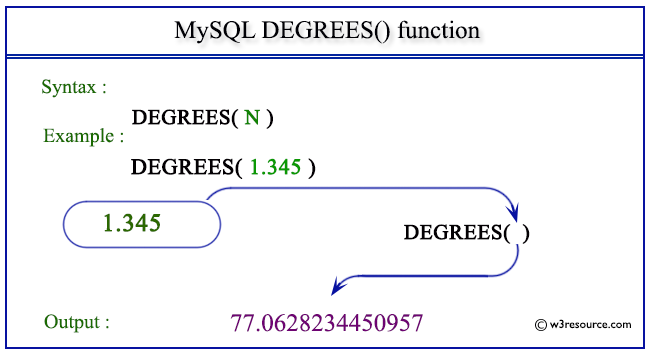# MySQL DEGREES() function

## DEGREES() function

MySQL DEGREES() converts the value in radians which is specified as the argument, to degrees.

Syntax:

```DEGREES(N);
```

Argument

Name Description
N A number in radians whose value in degrees is to be retrieved.

Syntax Diagram:MySQL Version: 5.6

Pictorial presentation of MySQL DEGREES() functionExample of MySQL DEGREES() function

Code:

``````SELECT DEGREES(1.345);
```
```

Explanation

The above MySQL statement will return the value of 1.345 in degrees.

Sample Output:

```mysql> SELECT DEGREES(1.345);
+------------------+
| DEGREES(1.345)   |
+------------------+
| 77.0628234450957 |
+------------------+
1 row in set (0.03 sec)
```

Example: degrees() with PI()

Code:

``````SELECT DEGREES(PI());
```
```

Explanation

The above MySQL statement will convert the radians value of PI() in degrees.

Sample Output:

```mysql> SELECT DEGREES(PI());
+---------------+
| DEGREES(PI()) |
+---------------+
|           180 |
+---------------+
1 row in set (0.00 sec)
```

All Mathematical FunctionsPrevious: CRC32()
Next: DIV

﻿